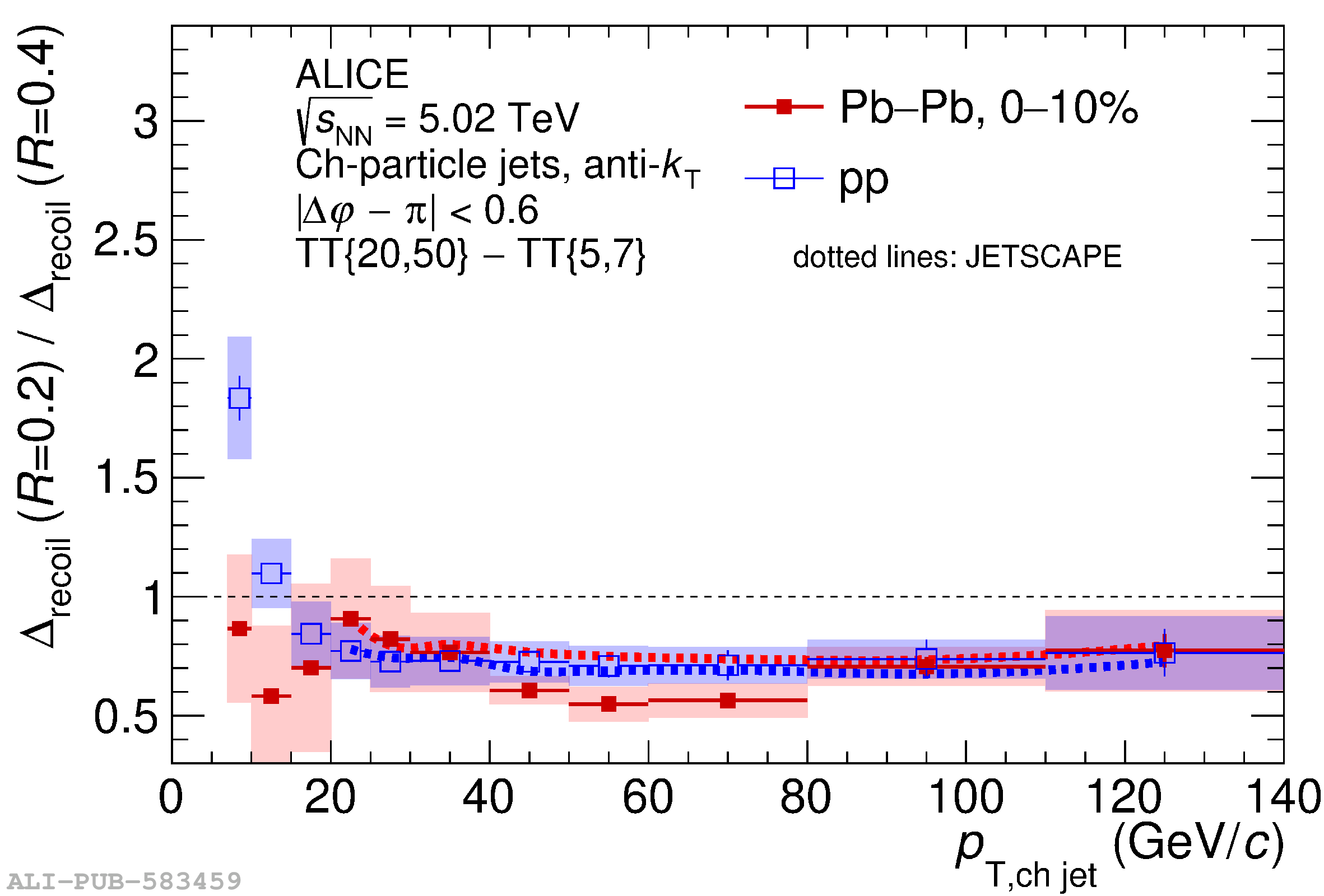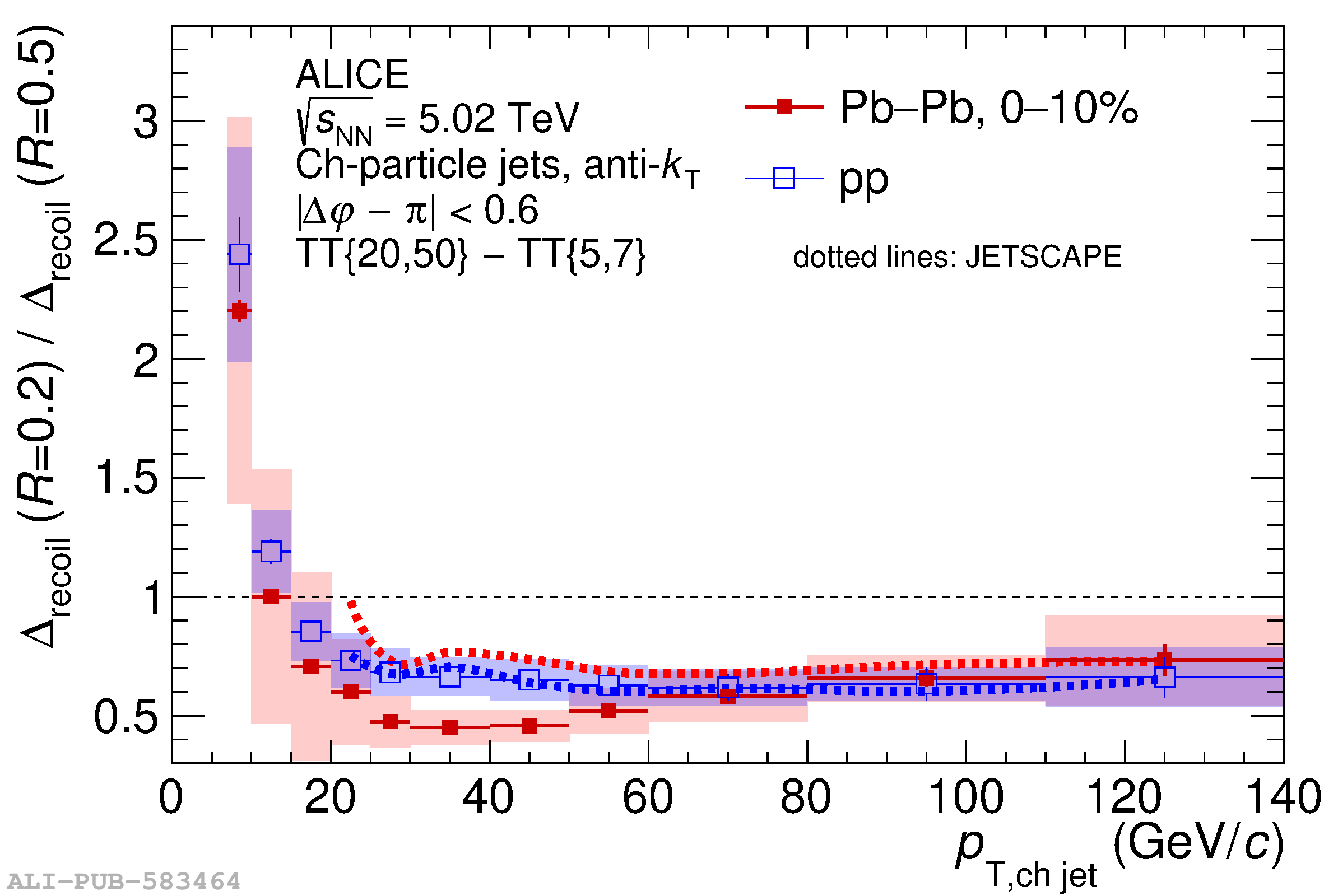# Figure 18

 Ratio of \DrecoilpTch\ distributions with different \rr\ for \PbPb\ collisions at $\sqrtsNN=5.02$ TeV from the data in Fig. \ref{fig:PbPb_Drecoil_pT}, together with the ratios for \pp\ collisions from Fig. \ref{fig:RRatios-pp}, for $\rr=0.2/\rr=0.4$ (left) and $\rr=0.2/\rr=0.5$ (right). The uncertainty in the ratio takes into account the correlation of uncertainties between numerator and denominator. JETSCAPE calculations for \pp\ and \PbPb\ collisions are also shown.